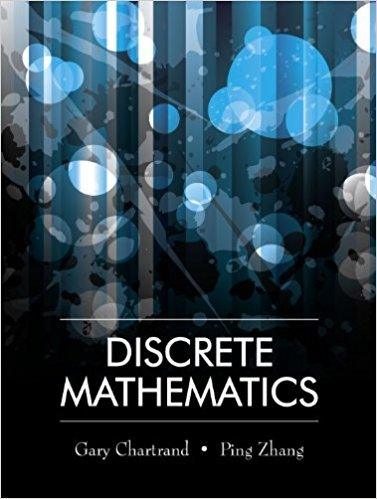×
×

# (a) Write a table that gives the different assignments of truth values for threeISBN: 9781577667308 385

## Solution for problem 1 Chapter 1.2

Discrete Mathematics | 1st Edition

• Textbook Solutions
• 2901 Step-by-step solutions solved by professors and subject experts
• Get 24/7 help from StudySoup virtual teaching assistantsDiscrete Mathematics | 1st Edition

4 5 1 360 Reviews
28
1
Problem 1

(a) Write a table that gives the different assignments of truth values for three statements P, Q and R if they are being considered simultaneously. (b) Maria has an interview for a job. For the statements P, Q and R below, write a sentence that corresponds to each row in the table in (a). P : Maria prepared for the interview. Q : Maria did well on the interview. R : Maria got the job.

Step-by-Step Solution:
Step 1 of 3

\D ftiat.,tat*tajic," @X\ry ctaslr-\$ (xr J {rrt^ d."nt^ { 3) =I...

Step 2 of 3

Step 3 of 3

##### ISBN: 9781577667308

The answer to “(a) Write a table that gives the different assignments of truth values for three statements P, Q and R if they are being considered simultaneously. (b) Maria has an interview for a job. For the statements P, Q and R below, write a sentence that corresponds to each row in the table in (a). P : Maria prepared for the interview. Q : Maria did well on the interview. R : Maria got the job.” is broken down into a number of easy to follow steps, and 75 words. Discrete Mathematics was written by and is associated to the ISBN: 9781577667308. This full solution covers the following key subjects: . This expansive textbook survival guide covers 79 chapters, and 1714 solutions. This textbook survival guide was created for the textbook: Discrete Mathematics, edition: 1. The full step-by-step solution to problem: 1 from chapter: 1.2 was answered by , our top Math solution expert on 03/13/18, 07:11PM. Since the solution to 1 from 1.2 chapter was answered, more than 241 students have viewed the full step-by-step answer.

Unlock Textbook Solution